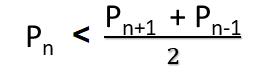# Check if N is a Weak Prime number or not

• Last Updated : 18 Mar, 2021

Given a positive integer N, the task is to check if N is a weak Prime or not.

In number theory, a weak prime is a prime number that is less than the arithmetic mean of nearest prime numbers i.e next and previous prime numbers.
First few weak prime numbers are 3, 7, 13, 19, 23, 31, 43, 47, 61, …
A weak prime Pn can be represented as-where n is its index in the ordered set of prime numbers.

Examples:

Input: N = 13
Output: Yes
13 is 6th prime number, the arithmetic mean of 5th and 7th prime number i.e. 11 and 17 is 14.
13 is less than 14 so 13 is a weak prime.

Input: N = 11
Output: No

Approach:

• If N is not a prime number or it is the first prime number i.e. 2 then print No.
• Else find the primes closest to N (one on the left and one on the right) and store their arithmetic mean in mean.
• If N < mean then print Yes.
• Else print No.

Below is the implementation of the above approach:

## C++14

 `// C++ program to check``// if a given number is weak prime``#include ``using` `namespace` `std;` `// Utility function to check``// if a number is prime or not``bool` `isPrime(``int` `n)``{``    ` `    ``// Corner cases``    ``if` `(n <= 1)``        ``return` `false``;``    ``if` `(n <= 3)``        ``return` `true``;` `    ``// This is checked so that we can skip``    ``// middle five numbers in below loop``    ``if` `(n % 2 == 0 || n % 3 == 0)``        ``return` `false``;` `    ``for``(``int` `i = 5; i * i <= n; i = i + 6)``        ``if` `(n % i == 0 || n % (i + 2) == 0)``            ``return` `false``;` `    ``return` `true``;``}` `// Function that returns true``// if n is a weak prime``bool` `isWeakPrime(``int` `n)``{``    ` `    ``// If n is not a prime number or``    ``// n is the first prime then return false``    ``if` `(!isPrime(n) || n == 2)``        ``return` `false``;` `    ``// Initialize previous_prime to n - 1``    ``// and next_prime to n + 1``    ``int` `previous_prime = n - 1;``    ``int` `next_prime = n + 1;` `    ``// Find next prime number``    ``while` `(!isPrime(next_prime))``        ``next_prime++;` `    ``// Find previous prime number``    ``while` `(!isPrime(previous_prime))``        ``previous_prime--;` `    ``// Arithmetic mean``    ``int` `mean = (previous_prime +``                ``next_prime) / 2;` `    ``// If n is a weak prime``    ``if` `(n < mean)``        ``return` `true``;``    ``else``        ``return` `false``;``}` `// Driver code``int` `main()``{``    ``int` `n = 13;` `    ``if` `(isWeakPrime(n))``        ``cout << ``"Yes"``;``    ``else``        ``cout << ``"No"``;` `    ``return` `0;``}` `// This code is contributed by himanshu77`

## Java

 `// Java program to check``// if a given number is weak prime``import` `java.util.*;``class` `GFG{` `// Utility function to check``// if a number is prime or not``static` `boolean` `isPrime(``int` `n)``{``    ``// Corner cases``    ``if` `(n <= ``1``)``        ``return` `false``;``    ``if` `(n <= ``3``)``        ``return` `true``;` `    ``// This is checked so that we can skip``    ``// middle five numbers in below loop``    ``if` `(n % ``2` `== ``0` `|| n % ``3` `== ``0``)``        ``return` `false``;` `    ``for` `(``int` `i = ``5``; i * i <= n; i = i + ``6``)``        ``if` `(n % i == ``0` `|| n % (i + ``2``) == ``0``)``            ``return` `false``;` `    ``return` `true``;``}` `// Function that returns true``// if n is a weak prime``static` `boolean` `isWeakPrime(``int` `n)``{``    ``// If n is not a prime number or``    ``// n is the first prime then return false``    ``if` `(!isPrime(n) || n == ``2``)``        ``return` `false``;` `    ``// Initialize previous_prime to n - 1``    ``// and next_prime to n + 1``    ``int` `previous_prime = n - ``1``;``    ``int` `next_prime = n + ``1``;` `    ``// Find next prime number``    ``while` `(!isPrime(next_prime))``        ``next_prime++;` `    ``// Find previous prime number``    ``while` `(!isPrime(previous_prime))``        ``previous_prime--;` `    ``// Arithmetic mean``    ``int` `mean = (previous_prime +``                ``next_prime) / ``2``;` `    ``// If n is a weak prime``    ``if` `(n < mean)``        ``return` `true``;``    ``else``        ``return` `false``;``}` `// Driver code``public` `static` `void` `main(String args[])``{``    ``int` `n = ``13``;` `    ``if` `(isWeakPrime(n))``        ``System.out.print(``"Yes"``);``    ``else``        ``System.out.print(``"No"``);``}``}` `// This code is contributed by Code_Mech`

## Python3

 `# Python3 program to check if a given``# number is weak prime` `# Utility function to check``# if a number is prime or not``def` `isPrime(n):``    ` `    ``# Corner cases``    ``if` `(n <``=` `1``):``        ``return` `False``    ``if` `(n <``=` `3``):``        ``return` `True` `    ``# This is checked so that we can skip``    ``# middle five numbers in below loop``    ``if` `(n ``%` `2` `=``=` `0` `or` `n ``%` `3` `=``=` `0``):``        ``return` `False` `    ``i ``=` `5``    ``while` `(i ``*` `i <``=` `n):``        ``if` `(n ``%` `i ``=``=` `0` `or` `n ``%` `(i ``+` `2``) ``=``=` `0``):``            ``return` `False``        ``i ``=` `i ``+` `6` `    ``return` `True` `# Function that returns true``# if n is a weak prime``def` `isWeakPrime(n):` `    ``# declaring variables as global``    ``global` `next_prime, previous_prime` `    ``# If n is not a prime number or n is``    ``# the first prime then return false``    ``if``(``not` `isPrime(n) ``or` `n ``=``=` `2``):``        ``return` `False` `    ``# Initialize previous_prime to n - 1``    ``# and next_prime to n + 1``    ``previous_prime ``=` `n ``-` `1``    ``next_prime ``=` `n ``+` `1` `    ``# Find next prime number``    ``while``(``not` `isPrime(next_prime)):``        ``next_prime ``+``=` `1` `    ``# Find previous prime number``    ``while` `(``not` `isPrime(previous_prime)):``        ``previous_prime ``-``=` `1` `    ``# Arithmetic mean``    ``mean ``=` `(previous_prime ``+` `next_prime) ``/``/` `2` `    ``# If n is a weak prime``    ``if``(n < mean):``        ``return` `True``    ``else``:``        ``return` `False` `# Driver code``if` `__name__ ``=``=` `'__main__'``:` `    ``n ``=` `13` `    ``if``(isWeakPrime(n)):``        ``print``(``"Yes"``)``    ``else``:``        ``print``(``"No"``)` `# This code is contributed by Shivam Singh`

## C#

 `// C# program to check if a given number is weak prime``using` `System;``class` `GFG {` `    ``// Utility function to check``    ``// if a number is prime or not``    ``static` `bool` `isPrime(``int` `n)``    ``{``        ``// Corner cases``        ``if` `(n <= 1)``            ``return` `false``;``        ``if` `(n <= 3)``            ``return` `true``;` `        ``// This is checked so that we can skip``        ``// middle five numbers in below loop``        ``if` `(n % 2 == 0 || n % 3 == 0)``            ``return` `false``;` `        ``for` `(``int` `i = 5; i * i <= n; i = i + 6)``            ``if` `(n % i == 0 || n % (i + 2) == 0)``                ``return` `false``;` `        ``return` `true``;``    ``}` `    ``// Function that returns true``    ``// if n is a weak prime``    ``static` `bool` `isWeakPrime(``int` `n)``    ``{``        ``// If n is not a prime number or``        ``// n is the first prime then return false``        ``if` `(!isPrime(n) || n == 2)``            ``return` `false``;` `        ``// Initialize previous_prime to n - 1``        ``// and next_prime to n + 1``        ``int` `previous_prime = n - 1;``        ``int` `next_prime = n + 1;` `        ``// Find next prime number``        ``while` `(!isPrime(next_prime))``            ``next_prime++;` `        ``// Find previous prime number``        ``while` `(!isPrime(previous_prime))``            ``previous_prime--;` `        ``// Arithmetic mean``        ``int` `mean = (previous_prime``                    ``+ next_prime)``                   ``/ 2;` `        ``// If n is a weak prime``        ``if` `(n < mean)``            ``return` `true``;``        ``else``            ``return` `false``;``    ``}` `    ``// Driver code``    ``public` `static` `void` `Main()``    ``{``        ``int` `n = 13;` `        ``if` `(isWeakPrime(n))``            ``Console.WriteLine(``"Yes"``);``        ``else``            ``Console.WriteLine(``"No"``);``    ``}``}`

## Javascript

 ``

Output:

`Yes`

My Personal Notes arrow_drop_up